Polygons

From DT Online

Description

Drawing regular Polygons is now most easily accomplished using modern drafting aids and Computer Aided Design tools. But there are occasions when it is useful to have an understanding of some basic constructions using just rule and compasses (or pegs and pieces of string). Examples might include large scale work for stage sets or carnival floats, or on-site work for buildings, playgrounds, sports fields and gardens.

The following examples are limited to constructions of regular polygons. Irregular polygon construction may be achieved using a process of triangulation, vectors, coordinates or plotting points on a matrix for example. Some other polygons (e.g. Cyclic Quadlitateral) have particular properties which can be helpful to know when constructing them.

See also the YouTube video All the possible polygons!. This shows how all regular polygons can be constructed using classical geometry techniques

Note: Some constructions on the YouTube video may be different to those described below - there are several methods of construction possible for most regular polygons.

Constructions
 Equilateral Triangle Draw a line of length equal to the length of side required. From each end of the line, strike arcs using the line length as radius. Where they intersect will provide the top apex of the required triangle.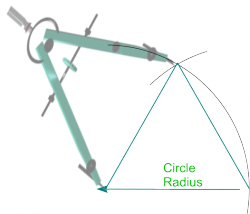Square Draw a line of length equal to the length of side required. From one end of the line construct a perpendicular - (see Basic Constructions). Set the line length as a radius and strike an arc along the perpendicular to find the second side of the square. Keep the same radius and strike arcs from the ends of the two adjacent sides as shown Joining to where the two arcs intersect provides the remaining two sides.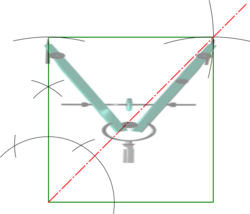Pentagon Draw a line of length equal to the length of side required and construct its perpendicular bisector - (see Basic Constructions). Draw a semi-circle at one end of the line and divide its circumference into as many equal parts as there are polygon sides required (5 in this case) The line connecting the semi-circle centre to the 2nd point is a side of the required polygon (pentagon in this case). Construct the perpendicular bisector of this second side to intersect with that drawn for the base line. Where the two bisectors intersect is the centre for a circumscribing circle to the required polygon. Draw the circle and step off the required number of sides. Note: Polygons with odd numbered sides have an apex at top centre and this can be used for greater accuracy as an alternative starting point, or as check, when stepping off sides round the circle . Note: This construction may be used for other polygons by stepping off an appropriate number of sides around the semi-circle - but in all cases always start by drawing a line through the 2nd division to establish the circumscribing circle centre.Hexagon Draw a line of length equal to the length of side required and construct an equilateral triangle on this as the base (see above) Set radius to length of side and draw the circumscribing circle for the hexagon from the top apex of the triangle. With same radius set, step off sides around the circle as shown. Note: Polygons with even numbers of sides have diagonals parallel to the sides and this can be used as a check on accuracyHeptagon (aka Septagon) Draw a line of length equal to the length of side required and construct its perpendicular bisector - (see Basic Constructions). From one end of the base line construct 450 and 600 angles. Where these to angles cross the vertical centre line would mark the centres of circumscribing circles for squares and hexagons respectively Bisect between these two centres. Note: A circle centred here would circumscribe a pentagon and provides an alternative construction. Set radius to half the distance between c4 and c6 and step off above c6 to create c7 - the centre of a circle which circumscribes a Heptagon (aka Septagon) Set radius to the length of side and step off round the circle.Octagon Draw a line of length equal to the length of side required and draw a semi-circle from each end Use the semi-circle arcs to construct perpendiculars from each end of the line - (see Basic Constructions). Bisect the exterior angles at the base to create 450 angles as shown. Where these bisectors cut the semi-circles are two sides of the octagon. Draw lines from the other end of the line through the top centre of the semi-circle - these will be parallel to the bisectors. Draw perpendiculars from where the 450 lines intersect the semi-circles up to their intersection with lines through the tops of the semi-circles - these are two vertical sides of the octagon. Complete the octagon by scribing lengths of sides from the ends of these vertical sides on to the first perpendiculars drawn as shown. Check the accuracy of all points by ensuring diagonals are parallel to sides.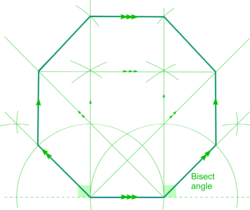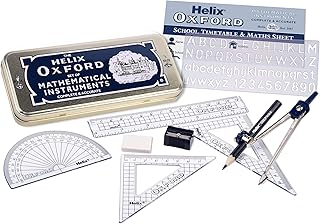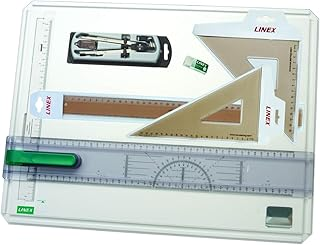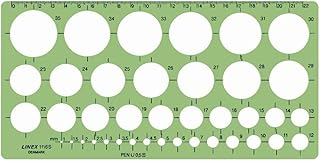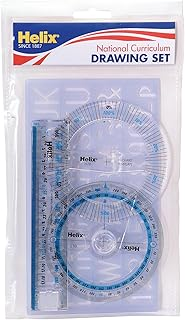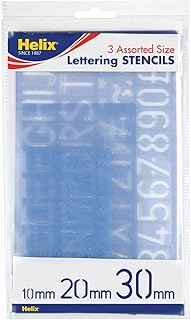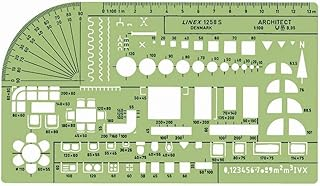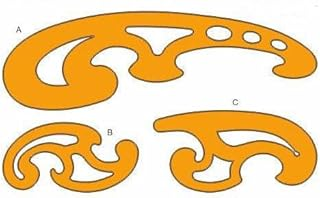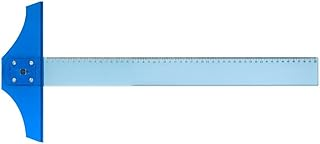Helix Oxford Maths SetLinex Student Saver BundleHelix Precision Plus Drawing SetPrimary Shape Template SetLinex Circle TemplateHelix National Curriculum Drawing SetHelix 3 Piece Stencil SetLinex Universal Architects TemplateFrench Curve Transparent TemplateT-Square